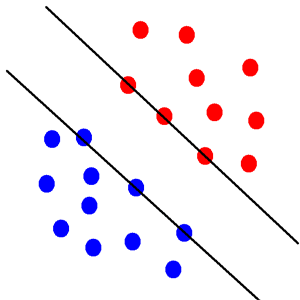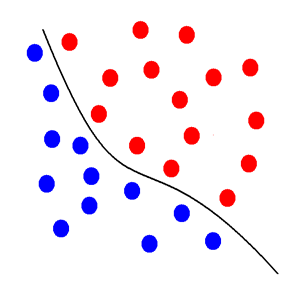## Support Vector Machines

1. Intro

Support Vector Machines (SVM), like artificial neural networks come from the computational theory of learning.  They have gained popularity recently as an alternative to ANN partly because statisticians better understand the theory behind them and because the computational time can be much shorter than with a ANN. They do not model interactions are effectively as ANN.

2. Overview

Support Vector Machines work by using non-linear transformation of the data elements to minimize the errors. The input space is transformed to the feature space using a kernel method. Kernel functions are the hearts to integral transformations which are used to map functions to new domains that are easier to solve. They are called Support Vector Machines because support vectors are used separate the transformed variables. Below are two illustrations of how a SVM’s transformation of the input space resulting better fitting models:By using non-linear transformation of the input space the support vectors can find the optimal solution where before there were no or multiple solutions. Like with the ANN, supervised SVM is used to forecast and an unsupervised SVM is used for classification.

3. SVM Types

There are four main types of SVM.  The central differences between each is the training function used and whether it is a classification or regression model.  As with any technique, it is advisable to use more than one type to see how the fit changes.

a) Regression models

1) Epsilon-SVR

With epsilon-SVMs the cost is zero when the predicted is close to the actual value.  This is to correct for noise.

2) NU-SVR
A non-linear loss function is used with NVM. This can lead to over fitting and long computation time.

b) Classification model

1) C-SVM

The complexity (the penalty factor) is constant in C-SVM.

2) NU-SVR
A non-linear loss function is used with NVM.

c) Distribution estimationO

One-class SVM are used to estimate distributions.

4. Kernel Methods

The choice of kernel method can have dramatic effects on the outcome of the model. There are a variety of different kernel methods below are the most commonly used for SVM.

a) Linear

Linear kernel methods only do a linear transformation of the input space.b) Radial Basis Function RBF

The most commonly used kernel type; the RBF provides a non-linear transformation of the input space.c) Polynomial

The polynomial kernel also provides a non-linear transformation of the input space.  Its values can go to infinity or zero in some circumstances.d) Sigmoid

The sigmoid kernel is a special case of the RBF kernel and provides a non-linear transformation of the input space.  Because it is a special case it is less flexible than the RBF type.